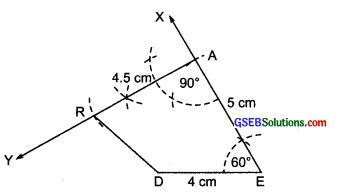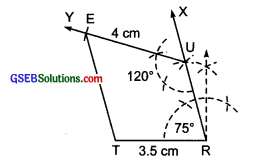# GSEB Solutions Class 8 Maths Chapter 4 Practical Geometry Ex 4.4

Gujarat Board GSEB Textbook Solutions Class 8 Maths Chapter 4 Practical Geometry Ex 4.4 Textbook Questions and Answers.

## Gujarat Board Textbook Solutions Class 8 Maths Chapter 4 Practical Geometry Ex 4.4

Question 1.
DEAR
DE = 4 cm
EA = 5 cm
AR = 4.5 cm
∠E = 60°
∠A = 90°TRUE
TR = 3.5 cm
RU = 3 cm
UE = 4 cm
∠R = 75°
∠U= 120°
Solution:
(i) Steps of construction:
I. Draw a line segment DE = 4 cm.
II. At E, draw $$\overrightarrow { EX }$$ such that ∠DEX = 60°.
III. From $$\overrightarrow { EX }$$, cut-off EA = 5 cm.
IV. At A, draw ray $$\overrightarrow { AY }$$ such that ∠EAY = 90°.
V. From $$\overrightarrow { EY }$$, cut-off AR = 4.5 cm.
VI. Join R and D.Thus, DEAR is the required quadrilateral.(ii) Steps of construction:I. Draw a line segment $$\overrightarrow { TR }$$ =3.5 cm.
II. At R, draw a ray $$\overrightarrow { RX }$$ such that ∠TRX = 15°.
III. From $$\overrightarrow { RX }$$ , cut-off RU = 3 cm.
IV. At U, draw $$\overrightarrow { UY }$$ such that ∠RUY = 120°
V From UY, cut-off $$\overrightarrow { UE }$$ = 4 cm.
VI. Join E and T.
Thus, TRUE is the required quadrilateral.